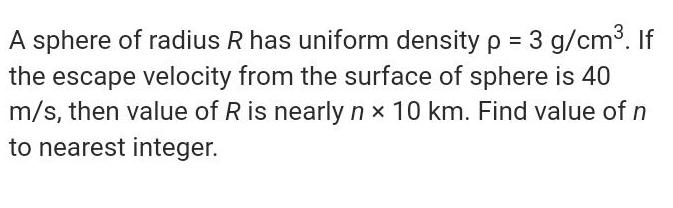Question:

# A sphere of radius R has uniform density p = 3 g/cm³. If the

Last updated: 7/10/2022A sphere of radius R has uniform density p = 3 g/cm³. If the escape velocity from the surface of sphere is 40 m/s, then value of R is nearly nx 10 km. Find value of n to nearest integer.